Assume that light of wavelength 7000 Angstrom is coming from a star. The limit of resolution (in radian) of a telescope whose objective has a diameter of 244 cm, will be

1.

2.

3.

4.

Concept Questions :-

Resolution of Optical devices
High Yielding Test Series + Question Bank - NEET 2020

Difficulty Level:

Which of the following is not due to the total internal reflection of light

1.  Brilliance of diamond

2.  Mirage on hot summer days

3.  Working of optional fiber

4.  formation of rainbow

Concept Questions :-

Total internal reflection
High Yielding Test Series + Question Bank - NEET 2020

Difficulty Level:

A ray of light incident on an equilateral prism at grazing incidence emerges from the prism at grazing emergence. Refractive index of the prism is

(1) 1.5

(2) 1.8

(3) 1.33

(4) 2

Concept Questions :-

Total internal reflection
High Yielding Test Series + Question Bank - NEET 2020

Difficulty Level:

Absolute refractive indices of glass and water are 3/2 and 4/3. The ratio of velocity of light in glass and water will be

1.  4:3

2.  8:7

3.  8:9

4.  3:4

Concept Questions :-

Refraction at plane surface
High Yielding Test Series + Question Bank - NEET 2020

Difficulty Level:

A thin equiconvex lens of power P is cut into three parts A, B, and C as shown in the figure. If  are powers of the three parts respectively, then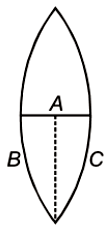1.

2.

3.

4.

Concept Questions :-

Lenses
High Yielding Test Series + Question Bank - NEET 2020

Difficulty Level:

A light beam containing red, yellow and blue color, falls on the interface of glass air as shown. If the yellow light is just totally internally reflected, then emergent ray in air contains-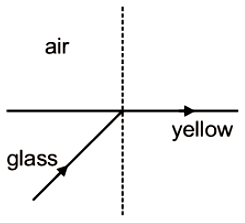1.  Both red and blue color

2.  Only red color

3.  Only blue color

4.  No color

Concept Questions :-

Total internal reflection
High Yielding Test Series + Question Bank - NEET 2020

Difficulty Level:

Focal lengths of objective and eyepiece of a compound microscope are 2 cm and 6.25 cm respectively. An object AB is placed at a distance of 2.5 cm from the objective which forms the image B'A' as shown in the figure. Maximum magnifying power in this case is :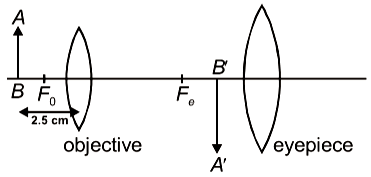1.  10

2.  20

3.  5

4.  25

Concept Questions :-

Simple and compound microscope
High Yielding Test Series + Question Bank - NEET 2020

Difficulty Level:

Two identical equiconvex thin lenses each of focal lengths 20 cm, made of material of refractive index 1.5 are placed coaxially in contact as shown. Now, the space between them is filled with a liquid of refractive index 1.5. The equivalent power of this arrangement will be: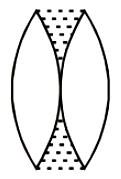1.  + 5D

2.  Zero

3.  + 2.5D

4.  + 0.5D

Concept Questions :-

Lensmakers' formula
High Yielding Test Series + Question Bank - NEET 2020

Difficulty Level:

The length of an astronomical telescope adjusted for parallel light is 90 cm. If the magnifying power of the telescope is 17, then the focal length of eyepiece and objective are respectively

1.  10 cm and 80 cm

2.  85 cm and 5 cm

3.  70 cm and 20 cm

4.  5 cm and 85 cm

Concept Questions :-

Telescope
High Yielding Test Series + Question Bank - NEET 2020

Difficulty Level:

A ray of light falls on a transparent sphere as shown in the figure. If the final ray emerges from the sphere parallel to the horizontal diameter, then calculate the refractive index of the sphere. Consider that the sphere is kept in the air.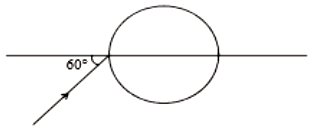1.

2.

3.

4.  $\sqrt{4/3}$

Concept Questions :-

Refraction at curved surface